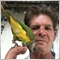175

```I do not know why just opening the purchase orders. Can someone help me?

//+------------------------------------------------------------------+
//|                                                      OneShot.mq4 |
//|                                                 Tarcísio Allyson |
//|                                             https://www.mql5.com |
//+------------------------------------------------------------------+
#property version   "1.00"
#property strict

// External variable
extern double LotSize = 0.1;
extern double TakeProfit = 5;
extern double StopLoss = 15;

extern int Slippage = 5;
extern int MagicNumber = 123;

extern int MAPeriodo = 7;
extern double Coeficiente = 0.14;

//Global variables

int SellTicket;
double UsePoint;
int UseSlippage;

//+------------------------------------------------------------------+
//| Expert initialization function                                   |
//+------------------------------------------------------------------+
int OnInit()
{
//---
Comment("EA Started");
UsePoint = PipPoint(Symbol());
UseSlippage = GetSlippage(Symbol(), Slippage);

return(0);

//---
return(INIT_SUCCEEDED);
}
//+------------------------------------------------------------------+
//| Expert deinitialization function                                 |
//+------------------------------------------------------------------+
void OnDeinit(const int reason)
{
//---

}
//+------------------------------------------------------------------+
//| Expert tick function                                             |
//+------------------------------------------------------------------+
void OnTick()
{
//---

// Envelope
double valorEnvBaixo = iEnvelopes(NULL,0,MAPeriodo,MODE_EMA,0,PRICE_CLOSE,Coeficiente,MODE_LOWER,0);
double valorEnvCima = iEnvelopes(NULL,0,MAPeriodo,MODE_EMA,0,PRICE_CLOSE,Coeficiente,MODE_UPPER,0);

if (Bid < valorEnvBaixo && BuyTicket == 0)
{

//Close order
if(OrderCloseTime() == 0 && SellTicket > 0)
{
double CloseLots = OrderLots();

bool Closed = OrderClose(SellTicket,CloseLots,ClosePrice,UseSlippage,Red);
}

// Calculate stop loss and take profit
if(StopLoss > 0) BuyStopLoss = OpenPrice - (StopLoss * UsePoint);
if(TakeProfit > 0) BuyTakeProfit = OpenPrice + (TakeProfit * UsePoint);

SellTicket = 0;
}

//Sell order
if (Bid > valorEnvCima && SellTicket == 0)
{
double OpenPrice = Bid;
double SellStopLoss;
double SellTakeProfit;

//Close order
if(OrderCloseTime() == 0 && BuyTicket > 0)
{
double CloseLots = OrderLots();
double ClosePrice = Bid;

}

Comment("Sell");
// Calculate stop loss and take profit
if(StopLoss > 0) SellStopLoss = OpenPrice - (StopLoss * UsePoint);
if(TakeProfit > 0) SellTakeProfit = OpenPrice + (TakeProfit * UsePoint);

// Open sell order
SellTicket = OrderSend(Symbol(),OP_SELL,LotSize,OpenPrice,UseSlippage,SellStopLoss,SellTakeProfit,"Sell Order",MagicNumber,0,Red);

}

}
//+------------------------------------------------------------------+

// Pip Point Fuction
double PipPoint(string Currency)
{
double CalcPoint;
int CalcDigits = MarketInfo(Currency, MODE_DIGITS);
if (CalcDigits == 2 || CalcDigits == 3) CalcPoint = 0.01;
else if (CalcDigits == 4 || CalcDigits == 5) CalcPoint = 0.0001;
return(CalcPoint);
}

// Get Slippage Function
int GetSlippage(string Currency, int SlippagePips)
{
double CalcSlippage;
int CalcDigits = MarketInfo(Currency,MODE_DIGITS);
if(CalcDigits == 2 || CalcDigits == 4) CalcSlippage = SlippagePips;
else if(CalcDigits == 3 || CalcDigits == 5) CalcSlippage = SlippagePips * 10;
return(CalcSlippage);
}

```445

northedan:

Just had a quick glance at the code - something is not right?

For a BUY TP is OpenPrice plus (+) Profit and SL is OpenPrice minus (-) StopLoss

For a SELL TP is OpenPrice minus (-)  Profit and SL is OpenPrice plus (+) StopLoss

Just check you code because it looks like you BUY and SELL is the same :)175

Barry Hendriks:

Just had a quick glance at the code - something is not right?

For a BUY TP is OpenPrice plus (+) Profit and SL is OpenPrice minus (-) StopLoss

For a SELL TP is OpenPrice minus (-)  Profit and SL is OpenPrice plus (+) StopLoss

Just check you code because it looks like you BUY and SELL is the same :)

Thank you!!! It's works.445

northedan:
Thank you!!! It's works.
I have been there - just cannot find the problem until someone else points to the problem :)175

Barry Hendriks:
I have been there - just cannot find the problem until someone else points to the problem :)
My EA opens a sales order, then buys, then sells, and then buys. Always following this sequence. He does not open orders followed by purchase or sale. I do not know how to solve this either. I believe I should create 2 EA for each operation.